Please Note: Blog posts are not selected, edited or screened by Seeking Alpha editors.

# Pros And Cons Of VaR Calculation Methods

|About:
Summary

Daily VaR is a widely used risk measure by Risk Management Floors around the world.

Different methods of calculating VaR yield very similar results.

Daily VaR is flawed as a standalone risk measure due to large skewness over shorter time intervals.

In this note I would like to discuss 1. The Pros and Cons of Different Methods of VaR Calculations, 2. The Difficulties of the Normal Distribution Assumption for Daily VaR Calculation. For the purpose of VaR discussion, I have constructed a hypothetical 1000 USD portfolio of 500 SPY (US Stock Market), 250 TLT (US Long Term Treasury),  & 250 EMB (Emerging Market Bond). All historical market data is from December 19, 2007 – June 27, 2019.

1. Pros and Cons of Different Methods of VaR Calculations

The three methods of VaR calculations I will discuss are Non Parametric, Parametric, and Semi Parametric. I will also calculate different methods of VaR, and compare the results.

Non Parametric or Historical approach take a sample of historical data, and use it to make assumptions about portfolio value at risk. Two important features of historical VaR are that the sample size of data must be sufficiently large, and future returns will be modelled on the results of past gains and losses. This is a relatively easy method to calculate VaR, but can be affected by more volatile historical returns skewing the data disproportionately. Below is a calculation of Historical VaR for our sample portfolio. The weights each component contribute to overall VaR are also given:

#\$MVaR

 0.008042791

#\$contribution

SPY           TLT           EMB

0.0064926568 -0.0003034561  0.0018535902

#\$pct_contrib_MVaR

SPY        TLT        EMB

0.8072642 -0.0377302  0.2304660

Parametric VaR make use of the Variance Covariance matrix, and we will cover a few different methods of Parametric VaR calculation. The simplest method we can calculate by hand in five steps using volatility estimates for our portfolio of SPY 14%, TLT 9%, EMB, 8%. I multiplied these volatilities by the square root of 1/262 to get daily volatility, and then filled in the diagonals of the matrix. Correlation coefficients were determined at SPY-TLT -0.43, SPY-EMB 0.25, & TLT-EMB -0.03. The matrix is multiplied by our position weights: 500 250 250, to find sigma, and then we transpose the sigma matrix and multiply again by our weights to find the variance. VaR is calculated at the 95 percentile using z-score 1.96. Our maximum daily loss of .9% is near to the .8% calculated from historical VaR.

This calculation is shown below.

SPY   TLT   EMB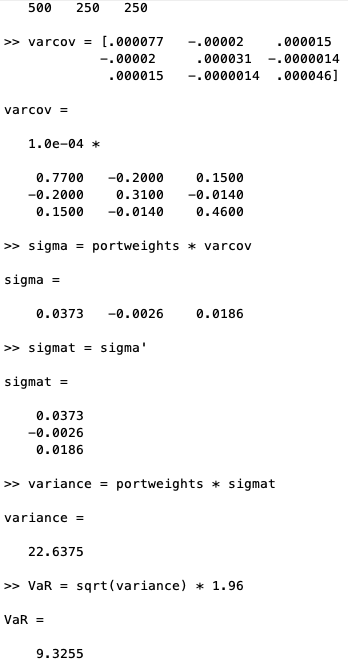Next, I calculated Parametric VaR with statistical software; Traditional (Hist), Gaussian, and Modified. Traditional parametric VaR was introduced by J.P. Morgan in 1994 and does a better job than non-parametric VaR of accounting for tails. However, it suffers if our portfolio varies widely from normality which is where Gaussian does a better job. Both Gaussian and traditional are efficient with a large data set that is normally distributed. They make use of measures of central tendency (Central Limit Theorem), which limits their effectiveness in highly skewed distributions. That is where Modified VaR is helpful. Modified uses Cornish Fisher math theorem which is an expansion model that creates a distribution that considers large skews. Below are the results of the three parametric daily VaR calculations to the 95 th percentile. Again, the results of 0.8% – 1% VaR are similar to what we have previously calculated.

SPY               TLT               EMB               Portfolio VaR (95%)

Hist   0.01875710   0.01475808   0.007014616   0.008238851

Gaus 0.02011211   0.01477953   0.010559099   0.010006260

Mod  0.01553630   0.01443925   0.007417950   0.008042791

* R Script for VaR calculation is given at end of article.

Another method of modelling VaR is through Monte Carlo simulation. Monte Carlo can be effective because it can include for sampling variability over a very large random sample. Monte Carlo can model non linearity effectively, and is useful for options and derivatives. This is because it can model path dependant payoff functions with regards to all inputs into the options model. Monte Carlo can be more involved to design. Another potential drawback is that it requires a volatility assumption. Below, I modelled a multivariate outcome by simulating the portfolio over 10,000 daily random incidents. I used the Cholesky Matrix for my volatility inputs. Even though our random sample was very large, our implied volatility is difficult to precisely predict for daily VaR predictions. Note that very few daily occurrences outside of three standard deviation occur, but this is more likely with SPY.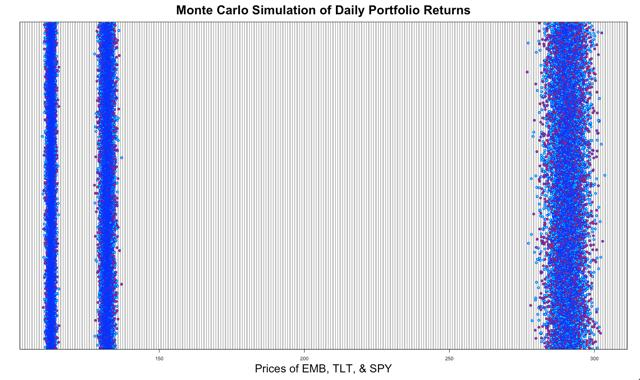The Semi Parametric model of VaR calculation relies on Extreme Value Theorem to deal with tail risk. The benefit is that no specific standard distribution is given emphasis over the whole distribution including the tails. The reason for this advantage is that the Extreme Value Theorem uses a Pareto Distribution to find the upper and lower bounds beyond a maximum extreme case scenario. Disadvantages are that proper mean may not be used and model variables such as stochastic volatility may be incorrect.

2. The Difficulties of the Normal Distribution Assumption for Daily VaR Calculation

I plotted the histograms and density of our portfolio in the charts below. In both histogram and density plot, daily distribution is very skinny or clustered around the mean (not normally distributed), whereas monthly returns a more typical normal distribution. In the daily histogram, there are also many values in the tails, and very randomly distributed. Over a larger time interval a normal distribution is more likely, but in the daily interval, even if it is only a 1/1500 probability, a stock can make an extremely large negative or positive skewed move. If our stock price is 100 with 20% volatility, we calculate with 99.7% confidence that movement will be up or down by 3.70 [3 * (100 * 20% * sqrt (1/262))]. If the stock moves more than this we may have had the wrong volatility estimate from the model, but we need large representative price distribution to determine. Therefore, over shorter periods Daily VaR may not follow normal tendencies.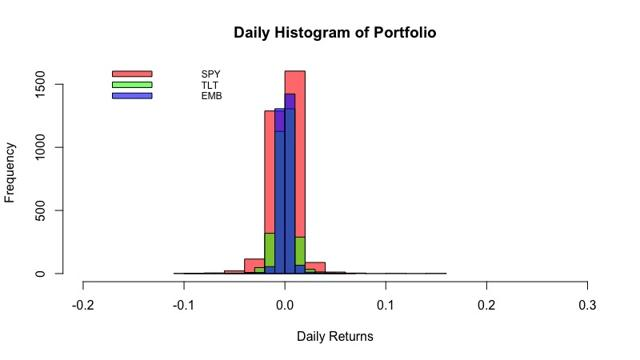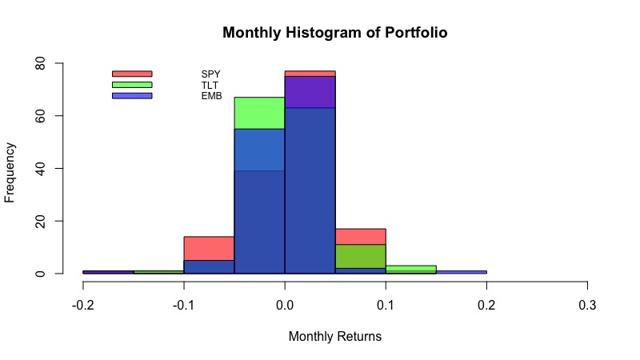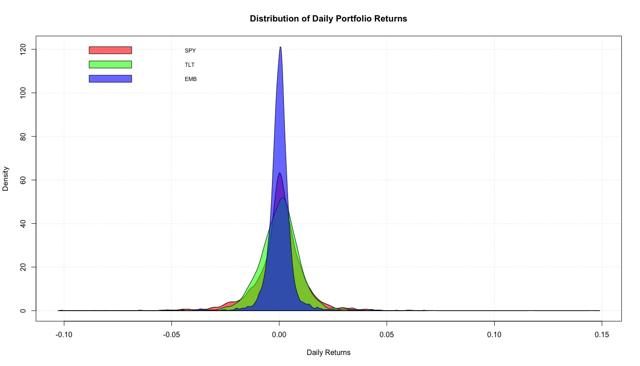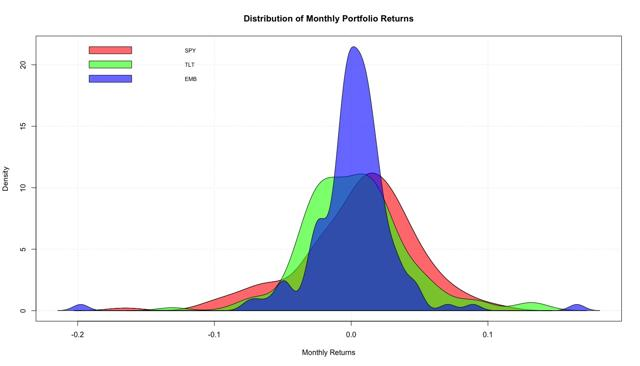################

#Packages#

################

install.packages("quantmod")

install.packages("PerformanceAnalytics")

library(quantmod)

library(PerformanceAnalytics)

#####################

#Portfolio VaR#

#####################

SPY <- Ad(getSymbols("SPY", from = "2007-12-19", auto.assign = F ))

TLT <- Ad(getSymbols("TLT", from = "2007-12-19", auto.assign = F ))

EMB <- Ad(getSymbols("EMB", from = "2007-12-19", auto.assign = F ))

Port.Adjusted <- merge(SPY,TLT,EMB)

Port.Returns <- na.omit(ROC(Port.Adjusted, type = "discrete"))

Weights <- c(0.5, 0.25, 0.25)

VaR.Hist <- VaR(Port.Returns, p=0.95, weights = NULL, portfolio_method = "single", method = "historical")

VaR.Gaus <- VaR(Port.Returns, p=0.95, weights = NULL, portfolio_method = "single", method = "gaussian")

VaR.Mod <- VaR(Port.Returns, p=0.95, weights = NULL, portfolio_method = "single", method = "modified")

VaR.All <- rbind(VaR.Hist,VaR.Gaus,VaR.Mod)

rownames(VaR.All) <- c("Hist", "Gaus", "Mod")

PortVaR.Hist <- VaR(Port.Returns, p=0.95, weights = Weights, portfolio_method = "component", method = "historical")\$hVaR

PortVaR.Gaus <- VaR(Port.Returns, p=0.95, weights = Weights, portfolio_method = "component", method = "gaussian")\$VaR

PortVaR.Mod <- VaR(Port.Returns, p=0.95, weights = Weights, portfolio_method = "component", method = "modified")\$MVaR

Portfolio <- rbind(PortVaR.Hist,PortVaR.Gaus,PortVaR.Mod)

print(Portfolio)

Final.Var <- cbind(VaR.Alle,Portfolio)

print(Final.Var)

abs(Final.Var)

Disclosure: I/we have no positions in any stocks mentioned, and no plans to initiate any positions within the next 72 hours.